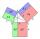# ABCD

AC= 40cm , angle DAB=38 , angle DCB=58 , angle DBC=90 , DB is perpendicular on AC , find BD and AD

Result

DB =  20.999 cm

#### Solution:

$DB=BD = 20.9994589142 \doteq 20.9995 = 20.999 \ \text { cm }$
$AD = 34.1087752861 \doteq 34.1088 = 34.109 \ \text { cm }$

Try calculation via our triangle calculator.

Leave us a comment of this math problem and its solution (i.e. if it is still somewhat unclear...):Be the first to comment!#### Following knowledge from mathematics are needed to solve this word math problem:

Do you want to convert length units? See also our right triangle calculator. Cosine rule uses trigonometric SAS triangle calculator. See also our trigonometric triangle calculator.

## Next similar math problems:

1. Four sides of trapezoidIn the trapezoid ABCD is |AB| = 73.6 mm; |BC| = 57 mm; |CD| = 60 mm; |AD| = 58.6 mm. Calculate the size of its interior angles.
2. A rhombusA rhombus has sides of length 10 cm, and the angle between two adjacent sides is 76 degrees. Find the length of the longer diagonal of the rhombus.
3. Triangle SASCalculate the area and perimeter of the triangle, if the two sides are 51 cm and 110 cm long and angle them clamped is 130 °.
4. Triangle ABCTriangle ABC has side lengths m-1, m-2, m-3. What has to be m to be triangle a) rectangular b) acute-angled?
5. Medians of isosceles triangleThe isosceles triangle has a base ABC |AB| = 16 cm and 10 cm long arm. What are the length of medians?
6. TriangleTriangle KLM is given by plane coordinates of vertices: K[11, -10] L[10, 12] M[1, 3]. Calculate its area and itsinterior angles.
7. Side cIn △ABC a=2, b=4 and ∠C=100°. Calculate length of the side c.
8. DiagonalsCalculate the length of the diagonals of the rhombus if its side is long 5 and one of its internal angle is 80°.
9. Diagonals in diamondIn the rhombus is given a = 160 cm, alpha = 60 degrees. Calculate the length of the diagonals.
10. Vector sumThe magnitude of the vector u is 12 and the magnitude of the vector v is 8. Angle between vectors is 61°. What is the magnitude of the vector u + v?
11. Greatest angleCalculate the greatest triangle angle with sides 197, 208, 299.
12. Three vectorsThe three forces whose amplitudes are in ratio 9:10:17 act in the plane at one point so that they are in balance. Determine the angles of the each two forces.
13. Angles by cosine lawCalculate the size of the angles of the triangle ABC, if it is given by: a = 3 cm; b = 5 cm; c = 7 cm (use the sine and cosine theorem).
14. Inner anglesThe inner angles of the triangle are 30°, 45° and 105° and its longest side is 10 cm. Calculate the length of the shortest side, write the result in cm up to two decimal places.
15. LawsFrom which law follows directly the validity of Pythagoras' theorem in the right triangle? ?
16. Triangle and its heightsCalculate the length of the sides of the triangle ABC, if va=5 cm, vb=7 cm and side b is 5 cm shorter than side a.
17. Heron backlawCalculate missing side in a triangle with sides 17 and 34 and area 275.